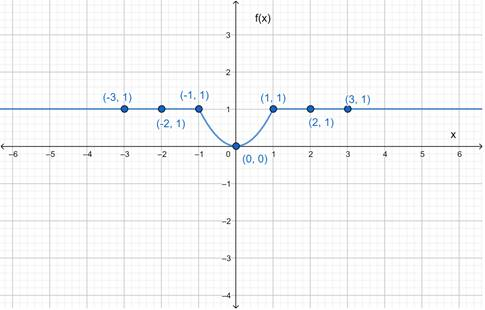# The graph of the given function.### Precalculus: Mathematics for Calcu...

6th Edition
Stewart + 5 others
Publisher: Cengage Learning
ISBN: 9780840068071### Precalculus: Mathematics for Calcu...

6th Edition
Stewart + 5 others
Publisher: Cengage Learning
ISBN: 9780840068071

#### Solutions

Chapter 2.2, Problem 44E
To determine

## To sketch : The graph of the given function.

Expert Solution

### Explanation of Solution

Given information :

f(x)={x2     if  |x|11       if  |x|>1

Graph :

|x|11x1

|x|>11>x>1

 x −3 −2 −1 0 1 2 3 f(x) 1 1 1 0 1 1 1This is the graph of the given piecewise defined equation.

Interpretation : The part of the graph to the left of x=1 coincides with the graph of f(x)=1 and the part of the graph to the right of x=1 coincides with the graph of f(x)=1 . The part of the graph between x=1 and x=1 coincides with f(x)=x2 .

### Have a homework question?

Subscribe to bartleby learn! Ask subject matter experts 30 homework questions each month. Plus, you’ll have access to millions of step-by-step textbook answers!